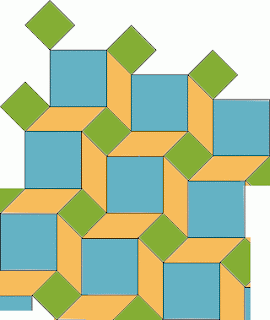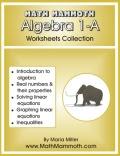## Posts

Showing posts from January, 2009

### Making the fractions in a proportion

"How do you know how to make the fractions in a proportion?
When making them, how do you know where each number goes making the fraction, like which ones go on top of the fraction?"

Well, actually you can choose which quantity will go on top; the proportion WILL work either way!

But, sometimes people are used to always putting certain quantity on top and certain on the bottom. For example, if the question is about speed and the unit is "miles per hour", that tells you that miles go on top, and hours on bottom, because "per" means division (the fraction line).

However, you could still solve the proportion by putting hours in the numerator of the fractions and miles in the denominator, and the calculation will turn out alright.

Or, if the question is about "dollars per pound", then dollars go to the numerator and pounds in the denominator.

Let's look at this problem for example:

A car drives on constant speed. It can go 80 miles in 90 minutes. How …

### Geometry problem: a tilingUPDATED!

Today I have a geometry problem for you.

Just yesterday I showed you a proportion problem and its solution.

(I want to insert a note here, especially for parents reading this.

Don't feel that you always need to use the problems I present here with your students. Instead, as a homeschooling parent, consider them part of your "math teacher training" that you never got. Reading through problems and their solutions will help you become a better math teacher. Consider yourself an apprentice observing a master completing a task, in this case mathematics problem solving.

It just takes you a minute or two to read this, think it through, try it - and understand (I hope). Your students' or child's book will have similar problems later on when it's time for geometry studies, but if you read these that I present, you will have learned more and can better tackle those problems at that time.)

OK, here we go. Look at the tiling below. Here you can see the tiling in real …

### A certain proportion

I need help explaining how to solve:

12 36
---- = ----
3w 63

Could you solve this if instead of 3w it was x?

Well you can do just that:

12 36
---- = ----
x 63

Now cross multiply and go about it as usual. The answer is x = 21.

But, x is actually 3w. So 3w = 21. So w = 7.

This changing of variables is a very often used "trick" in mathematics.

Alternate solution.

Upon examining the proportion, we notice both the numbers in the first fraction are divisible by 3, and both numbers in the second fraction are divisible by 9.

12 36
---- = ----
3w 63

So, we can write it as follows and simplify both fractions (the right and left sides):

3 * 4 9 * 4
------ = -------
3w 9 * 7

4 4
--- = ---
w 7
You can't find a simpler proportion than that, obviously w must equal 7.

### Free e-book: Number Stories of Long agoCatch if while you can! HomeschoolFreebie of the day offers TODAY a mathematics book called Number Stories of Long Ago . Tuesday January 20 only.

### AlcumusAlcumus is a brand new online math program - currently free - by the Art of Problem Solving folks. This program is specifically designed to provide a challenging enrichment program for gifted math students.

Alcumus interacts with the student and adapts to his or her level. Currently, the program contains over 1100 problems with fully worked-out solutions and over 60 video lessons.

Anyone can join - whether you are just interested in exploring math a little further, or you have student who is training for some math contest, etc.

However, currently one can only join the program as part of a registered class. If you are a single person, this can be a problem. Fortunately, Denise at Let's Play Math has set up a class which is open to all interested homeschoolers and self-directed learners. Please click here to find out more about joining Alcumus as part of Denise's class. Otherwise, if you have a class, just go straight to Alcumus and join there.

### When will I ever need this (math)?We all know that youngsters often have this question in mind when they go on in their math studies: where or when will I ever need all this?

It's pretty easy and simple to find applications and real-life examples for the usage of the simple, elementary math, such as multiplication tables or decimals and percents. But when we go on to algebra and beyond, this question may be harder to answer.

Here are a few answers you can give as to why study algebra:
You need it in any occupational field that requires higher education, such as computer science, electronics, engineering, medicine (doctors), trade and commerce analysts, ALL scientists, etc. In short, if someone is even considering higher education, they should study algebra. You need algebra to take your SAT test or GED.
Algebra also lets you develop logical thinking and problem solving skills. It can increase your intelligence! Actually, studying any math topic can do that (even elementary math), if the mathematics is presented and …

### ExploreLearning open house

If you've been to ExploreLearning before, you know they offer interactive online simulations or demos for science and math, called Gizmos. These gizmos are pretty neat things!

Anyway, I'm just passing this on. They happen to have an OPEN HOUSE, in other words, a completely free 45-day trial. Use promo code OpenHouse09 to take advantage of this trial offer, which ends on January 21st.

a

### Math Contest at MathNotations

I was asked to pass on the word on this free TEAM-BASED math contest... geared towards middle/high school students. Teachers, take note!

Dave Marain from MathNotations has authored a Math Contest, which will take place on Tuesday February 3rd.

Students in Grades 7-12 may participate. Schools can register one team of up to 6 students to try the contest which will be different from most math competitions:
Team-based - students will have up to 90 minutes to complete 6 questions (although many teams could finish in 45 minutes)
Calculator permitted
Some multi-part questions and some open-ended questions requiring explanation a la MathNotations investigations
Emphasis on Algebra II although some questions are accessible to Middle SchoolersRegistration, delivery of questions and submission of official team answers/solutions all done via emailAll students receive a Certificate of Participation and top-scoring schools/students will receive a Certificate of MeritHere's the link to the info needed…

### Adding It Up: Helping Children Learn Mathematics

I wanted to pass on this book link to those of you who are interested in the pedagogy of mathematics: Adding It Up: Helping Children Learn Mathematics (2001) by Center for Education (CFE)

This book talks a lot about how the conceptual understanding of basic arithmetic develops in children during grades 1-8. It explains common misconceptions. The book cites a lot of research about how children learn addition, subtraction, multiplication, division, fractions, and proportions. It doesn't deal with geometry or measuring, because the authors decided to concentrate on "number" concepts.

All in all I think it's good basic reading for all of us who teach math. You can read it online by chapters. Here's a direct link to page 187 which starts dealing with single-digit addition. Here's a table comparing various addition and subtraction problem types. And here's a link to the discussion about single-dig…

### A little trick for square roots (mental math)

Someone sent me this little mental math trick for square roots. I liked it, didn't know it before, so here goes:
I read your suggestion for calculating square root without a calculator. I teach Math for Elementary Teachers and developmental math courses (algebra) to adults. I feel that the focus should be on understanding the number rather than an exercise in following a memorized algorithm. I suggest you have the student determine the pair of perfect squares the number falls between. For example, if finding the sqrt of 645, it falls between the sqrt of 625 which equals 25 and the sqrt of 676 which equals 26. So the sqrt of 645 has to be between 25 and 26. Where does it fall between? There are 50 numbers between 676 and 625. 645 is 20 numbers beyond 625, so 20/50 = 0.4
So the sqrt of 645 is very close to 25.4
This method provides the student with a process that improves their understanding of numbers without expecting them to memorize an algorithm, and it provides an answer to the ne…

### Free algebra worksheetsUsually algebra textbooks provide lots of problems to practice the algebraic concepts and techniques, but some of you may still benefit from resources for free (or mostly so) printable algebra worksheets. Please see the list below, which I've originally compiled for my HomeschoolMath.net site.

Algebra worksheets

Free worksheets for variable expressions
Worksheets for writing simple expressions with variables, when the expressions are given with words. For grades 6-8, pre-algebra, and algebra 1.
www.homeschoolmath.net/worksheets/variable_expressions.php

Free worksheets for evaluating expressions
Worksheets for evaluating simple expressions with variables, when the value of the variable(s) are given. For grades 6-8, pre-algebra, and algebra 1.
www.homeschoolmath.net/worksheets/evaluate_expressions.php

Free worksheets for linear equations
Worksheets for linear equations. Includes one-step, two-step, multi-step equations, variable on both sides, and more. For grades 6-9, pre-algebra, an…